# HC Verma solutions for Class 11, Class 12 Concepts of Physics Vol. 1 chapter 11 - Gravitation [Latest edition]

#### Chapters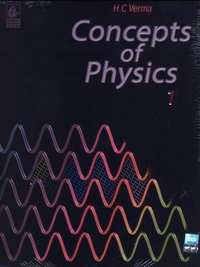## Solutions for Chapter 11: Gravitation

Below listed, you can find solutions for Chapter 11 of CBSE, Karnataka Board PUC HC Verma for Class 11, Class 12 Concepts of Physics Vol. 1.

Short Answers [Pages 223 - 224]

### HC Verma solutions for Class 11, Class 12 Concepts of Physics Vol. 1 Chapter 11 Gravitation Short Answers [Pages 223 - 224]

Short Answers | Q 1 | Page 223

Can two particles be in equilibrium under the action of their mutual gravitational force? Can three particles be? Can one of the three particles be?

Short Answers | Q 2 | Page 223

Is there any meaning of "Weight of the earth"?

Short Answers | Q 3 | Page 223

If heavier bodies are attracted more strongly by the earth, why don't they fall faster than the lighter bodies?

Short Answers | Q 4 | Page 223

Can you think of two particles which do not exert gravitational force on each other?

Short Answers | Q 5 | Page 223

The earth revolves round the sun because the sun attracts the earth. The sun also attracts the moon and this force is about twice as large as the attraction of the earth on the moon. Why does the moon not revolve round the sun? Or does it?

Short Answers | Q 6 | Page 223

At noon, the sun and the earth pull the objects on the earth's surface in opposite directions. At midnight, the sun and the earth pull these objects in same direction. Is the weight of an object, as measured by a spring balance on the earth's surface, more at midnight as compared to its weight at noon?

Short Answers | Q 7 | Page 223

An apple falls from a tree. An insect in the apple finds that the earth is falling towards it with an acceleration g. Who exerts the force needed to accelerate the earth with this acceleration g?

Short Answers | Q 8 | Page 223

Suppose the gravitational potential due to a small system is k/r2 at a distance r from it. What will be the gravitational field? Can you think of any such system? What happens if there were negative masses?

Short Answers | Q 9 | Page 223

The gravitational potential energy of a two-particle system is derived in this chapter as "U"=("Gm"_1"m"_2)/"r". Does it follow from this equation that the potential energy for $r = \infty$ must be zero? Can we choose the potential energy for $r = \infty$ to be 20 J and still use this formula? If no, what formula should be used to calculate the gravitational potential energy at separation r ?

Short Answers | Q 10 | Page 223

The weight of an object is more at the poles than at the equator. Is it beneficial to purchase goods at equator and sell them at the pole? Does it matter whether a spring balance is used or an equal-beam balance is used?

Short Answers | Q 11 | Page 223

The weight of a body at the poles is greater than the weight at the equator. Is it the actual weight or the apparent weight we are talking about? Does your answer depend on whether only the earth's rotation is taken into account or the flattening of the earth at the poles is also taken into account?

Short Answers | Q 12 | Page 224

If the radius of the earth decreases by 1% without changing its mass, will the acceleration due to gravity at the surface of the earth increase or decrease? If so, by what per cent?

Short Answers | Q 13 | Page 224

A nut becomes loose and gets detached from a satellite revolving around the earth. Will it land on the earth? If yes, where will it land? If no, how can an astronaut make it land on the earth?

Short Answers | Q 14 | Page 224

Is it necessary for the plane of the orbit of a satellite to pass through the centre of the earth?

Short Answers | Q 15 | Page 224

Consider earth satellites in circular orbits. A geostationary satellite must be at a height of about 36000 km from the earth's surface. Will any satellite moving at this height be a geostationary satellite? Will any satellite moving at this height have a time period of 24 hours?

Short Answers | Q 16 | Page 224

No part of India is situated on the equator. Is it possible to have a geostationary satellite which always remains over New Delhi?

Short Answers | Q 17 | Page 224

As the earth rotates about its axis, a person living in his house at the equator goes in a circular orbit of radius equal to the radius of the earth. Why does he/she not feel weightless as a satellite passenger does?

Short Answers | Q 18 | Page 224

Two satellites going in equatorial plane have almost same radii. As seen from the earth one moves from east one to west and the other from west to east. Will they have the same time period as seen from the earth? If not which one will have less time period?

Short Answers | Q 19 | Page 224

A spacecraft consumes more fuel in going from the earth to the moon than it takes for a return trip. Comment on this statement.

MCQ [Pages 224 - 225]

### HC Verma solutions for Class 11, Class 12 Concepts of Physics Vol. 1 Chapter 11 Gravitation MCQ [Pages 224 - 225]

MCQ | Q 1 | Page 224

The acceleration of moon with respect to earth is 0⋅0027 m s−2 and the acceleration of an apple falling on earth' surface is about 10 m s−2. Assume that the radius of the moon is one fourth of the earth's radius. If the moon is stopped for an instant and then released, it will fall towards the earth. The initial acceleration of the moon towards the earth will be

• 10 m s−2

• 0⋅0027 m s−2

• 6⋅4 m s−2

• 5⋅0 m s−2.

MCQ | Q 2 | Page 224

The acceleration of the moon just before it strikes the earth in the previous question is

• 10 m s−2

• 0⋅0027 m s−2

• 6⋅4 m s−2

• 5⋅0 m s−2

MCQ | Q 3 | Page 224

Suppose, the acceleration due to gravity at the earth's surface is 10 m s−2 and at the surface of Mars it is 4⋅0 m s−2. A 60 kg passenger goes from the earth to the Mars in a spaceship moving with a constant velocity. Neglect all other objects in the sky. Which part of the following figure best represents the weight (net gravitational force) of the passenger as a function of time?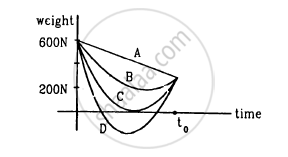• A

• B

• C

• D

MCQ | Q 4 | Page 224

Consider a planet in some solar system which has a mass double the mass of the earth and density equal to the average density of the earth. An object weighing W on the earth will weight

• W

• 2 W

• W/2

• 21/3 W at the planet.

MCQ | Q 5 | Page 224

If the acceleration due to gravity at the surface of the earth is g, the work done in slowly lifting a body of mass m from the earth's surface to a height R equal to the radius of the earth is

• $\frac{1}{2}mgR$

• $2mgR$

• $mgR$

• $\frac{1}{4}mgR$

MCQ | Q 6 | Page 224

A person brings a mass of 1 kg from infinity to a point A. Initially the mass was at rest but it moves at a speed of 2 m s −1 as it reaches A. The work done by the person on the mass is −3 J. The potential at A is

• −3 J kg−1

• −2 J kg−1

• −5 J kg−4

• none of these.

MCQ | Q 7 | Page 224

Let V and E be the gravitational potential and gravitational field at a distance r from the centre of a uniform spherical shell. Consider the following two statements :

(A) The plot of V against r is discontinuous.
(B) The plot of E against r is discontinuous.

• Both A and B are correct.

• A is correct but B is wrong.

• B is correct but A is wrong.

• Both A and B are wrong.

MCQ | Q 8 | Page 224

Let V and E represent the gravitational potential and field at a distance r from the centre of a uniform solid sphere. Consider the two statements:
(A) the plot of V against r is discontinuous.
(B) The plot of E against r is discontinuous.

• Both A and B are correct.

• A is correct but B is wrong.

• B is correct but A is wrong.

• Both A and B are wrong.

MCQ | Q 9 | Page 224

Take the effect of bulging of earth and its rotation in account. Consider the following statements :

(A) There are points outside the earth where the value of g is equal to its value at the equator.
(B) There are points outside the earth where the value of g is equal to its value at the poles.

• Both A and B are correct.

• A is correct but B is wrong.

• B is correct but A is wrong.

• Both A and B are wrong.

MCQ | Q 10 | Page 225

The time period of an earth-satellite in circular orbit is independent of

• the mass of the satellite

• none of them

• both of them.

MCQ | Q 11 | Page 225

The magnitude of gravitational potential energy of the moon-earth system is U with zero potential energy at infinite separation. The kinetic energy of the moon with respect to the earth is K.

• U < K

• U > K

• U = K

MCQ | Q 12 | Page 225

In the Following figure shows the elliptical path of a planet about the sun. The two shaded parts have equal area. If t1 and t2 be the time taken by the planet to go from a to b and from c to d respectively,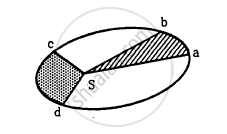• t1 < t2

• t1 = t2

• t1 > t2

• insufficient information to deduce the relation between t1 and t2

MCQ | Q 13 | Page 225

A person sitting in a chair in a satellite feels weightless because

• the earth does not attract the objects in a satellite

• the normal force by the chair on the person balances the earth's attraction

• the normal force is zero

• the person in satellite is not accelerated.

MCQ | Q 14 | Page 225

A body is suspended from a spring balance kept in a satellite. The reading of the balance is W1 when the satellite goes in an orbit of radius R and is W2 when it goes in an orbit of radius 2 −R.

• W1 = W2

• W1 < W2

• W1 > W2

• W≠ W2

MCQ | Q 15 | Page 225

The kinetic energy needed to project a body of mass m from the earth' surface to infinity is

• $\frac{1}{4}$mgR

• $\frac{1}{2}$mgR

• mgR

• 2 mgR

MCQ | Q 16 | Page 225

A particle is kept at rest at a distance R (earth's radius) above the earth's surface. The minimum speed with which it should be projected so that it does not return is

• $\sqrt{\frac{GM}{4R}}$

• $\sqrt{\frac{GM}{2R}}$

• $\sqrt{\frac{GM}{R}}$

• $\sqrt{\frac{2GM}{R}}$

MCQ | Q 17 | Page 225

A satellite is orbiting the earth close to its surface. A particle is to be projected from the satellite to just escape from the earth. The escape speed from the earth is ve. Its speed with respect to the satellite

• will be less than $\nu_e$

• will be more than $\nu_e$

• will be equal to $\nu_e$

• will depend on the direction of projection.

MCQ [Page 225]

### HC Verma solutions for Class 11, Class 12 Concepts of Physics Vol. 1 Chapter 11 Gravitation MCQ [Page 225]

MCQ | Q 1 | Page 225

Let V and E denote the gravitational potential and gravitational field at a point. It is possible to have

(a) $V = 0 \text { and }E = 0$

(b) $V = 0 \text { and } E \neq 0$

(c) $V \neq 0 \text { and }E = 0$

(d) $V \neq 0 \text { and }E \neq 0$

MCQ | Q 2 | Page 225

Inside a uniform spherical shell
(a) the gravitational potential is zero
(b) the gravitational field is zero
(c) the gravitational potential is same everywhere
(d) the gravitational field is same everywhere

MCQ | Q 3 | Page 225

A uniform spherical shell gradually shrinks maintaining its shape. The gravitational potential at the centre

• increases

• decreases

• remains constant

• oscillates

MCQ | Q 4 | Page 225

Consider a planet moving in an elliptical orbit round the sun. The work done on the planet by the gravitational force of the sun
(a) is zero in any small part of the orbit
(b) is zero in some parts of the orbit
(c) is zero in one complete revolution
(d) is zero in no part of the motion.

MCQ | Q 5 | Page 225

Two satellites A and B move round the earth in the same orbit. The mass of B is twice the mass of A.

• Speeds of A and B are equal.

• The potential energy of earth+A is same as that of earth+B.

• The kinetic energy of A and B are equal.

• The total energy of earth+A is same as that of earth+B.

MCQ | Q 6 | Page 225

Which of the following quantities remain constant in a planetary motion (consider elliptical orbits) as seen from the sun?

• Speed

• Angular speed

• Kinetic Energy

• Angular momentum.

Exercise [Pages 225 - 227]

### HC Verma solutions for Class 11, Class 12 Concepts of Physics Vol. 1 Chapter 11 Gravitation Exercise [Pages 225 - 227]

Exercise | Q 1 | Page 225

Two spherical balls of mass 10 kg each are placed 10 cm apart. Find the gravitational force of attraction between them.

Exercise | Q 2 | Page 225

Four particles having masses m, 2m, 3m and 4m are placed at the four corners of a square of edge a. Find the gravitational force acting on a particle of mass m placed at the centre.

Exercise | Q 3 | Page 226

Three equal masses m are placed at the three corners of an equilateral triangle of side a. Find the force exerted by this system on another particle of mass m placed at (a) the mid-point of a side, (b) at the centre of the triangle.

Exercise | Q 4 | Page 226

Three uniform spheres each having a mass M and radius a are kept in such a way that each touches the other two. Find the magnitude of the gravitational force on any of the spheres due to the other two.

Exercise | Q 5 | Page 226

Four particles of equal masses M move along a circle of radius R under the action of their mutual gravitational attraction. Find the speed of each particle.

Exercise | Q 6 | Page 226

Find the acceleration due to gravity of the moon at a point 1000 km above the moon's surface. The mass of the moon is 7.4 × 1022 kg and its radius is 1740 km.

Exercise | Q 7 | Page 226

Two small bodies of masses 10 kg and 20 kg are kept a distance 1.0 m apart and released. Assuming that only mutual gravitational forces are acting, find the speeds of the particles when the separation decreases to 0.5 m.

Exercise | Q 8 | Page 226

A semicircular wire has a length L and mass M. A particle of mass m is placed at the centre of the circle. Find the gravitational attraction on the particle due to the wire.

Exercise | Q 9 | Page 226

Derive an expression for the gravitational field due to a uniform rod of length L and mass M at a point on its perpendicular bisector at a distance d from the centre.

Exercise | Q 10 | Page 226

Two concentric spherical shells have masses M1, M2 and radii R1, R2 (R1 < R2). What is the force exerted by this system on a particle of mass m1 if it is placed at a distance (R1+ R2)/2 from the centre?

Exercise | Q 11 | Page 226

A tunnel is dug along a diameter of the earth. Find the force on a particle of mass m placed in the tunnel at a distance x from the centre.

Exercise | Q 12 | Page 226

A tunnel  is dug along a chord of the earth at a perpendicular distance R/2 from the earth's centre. The wall of the tunnel may be assumed to be frictionless. Find the force exerted by the wall on a particle of mass m when it is at a distance x from the centre of the tunnel.

Exercise | Q 13 | Page 226

A solid sphere of mass m and radius r is placed inside a hollow thin spherical shell of mass M and radius R as shown in the following figure . A particle of mass m' is placed on the line joining the two centres at a distance x from the point of contact of the sphere and the shell. Find the magnitude of the resultant gravitational force on this particle due to the sphere and the shell if (a) r < x < 2r, (b) 2r < x < 2R and (c) x > 2R.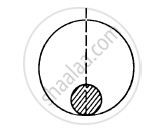Exercise | Q 14 | Page 226

A uniform metal sphere of radius a and mass M is surrounded by a thin uniform spherical shell of equal mass and radius 4a (In the following figure). The centre of the shell falls on the surface of the inner sphere. Find the gravitational field at the points P1 and P2 shown in the figure.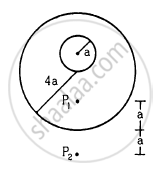Exercise | Q 15 | Page 226

A thin spherical shell having uniform density is cut in two parts by a plane and kept separated as shown in the following figure. The point A is the centre of the plane section of the first part and B is the centre of the plane section of the second part. Show that the gravitational field at A due to the first part is equal in magnitude to the gravitational field at B due to the second part.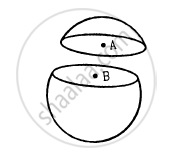Exercise | Q 16 | Page 226

Two small bodies of masses 2.00 kg and 4.00 kg are kept at rest at a separation of 2.0 m. Where should a particle of mass 0.10 kg be placed to experience no net gravitational force from these bodies? The particle is placed at this point. What is the gravitational potential energy of the system of three particles with usual reference level?

Exercise | Q 17 | Page 226

Three particles of mass m each are placed at the three corners of an equilateral triangle of side a. Find the work which should be done on this system to increase the sides of the triangle to 2a.

Exercise | Q 18 | Page 226

A particle of mass 100 g is kept on the surface of a uniform sphere of mass 10 kg and radius 10 cm. Find the work to be done against the gravitational force between them to take the particle away from the sphere.

Exercise | Q 19 | Page 226

The gravitational field in a region is given by

$\vec{E} = \left( 5 N {kg}^{- 1} \right) \vec{i} + \left( 12 N {kg}^{- 1} \right) \vec{j}$ . (a) Find the magnitude of the gravitational force acting on a particle of mass 2 kg placed at the origin. (b) Find the potential at the points (12 m, 0) and (0, 5 m) if the potential at the origin is taken to be zero. (c) Find the change in gravitational potential energy if a particle of mass 2 kg is taken from the origin to the point (12 m, 5 m). (d) Find the change in potential energy if the particle is taken from (12 m, 0) to (0, 5 m).

Exercise | Q 20 | Page 227

The gravitational potential in a region is given by V = 20 N kg−1 (x + y). (a) Show that the equation is dimensionally correct. (b) Find the gravitational field at the point (x, y). Leave your answer in terms of the unit vectors $\vec{i} , \vec{j} , \vec{k}$ . (c) Calculate the magnitude of the gravitational force on a particle of mass 500 g placed at the origin.

Exercise | Q 21 | Page 227

The gravitational field in a region is given by $E = \left( 2 \overrightarrow{i} + 3 \overrightarrow{j} \right) N {kg}^{- 1}$ . Show that no work is done by the gravitational field when a particle is moved on the line 3y + 2x = 5.

[Hint : If a line y = mx + c makes angle θ with the X-axis, m = tan θ.]

Exercise | Q 22 | Page 227

Find the height over the Earth's surface at which the weight of a body becomes half of its value at the surface.

Exercise | Q 23 | Page 227

What is the acceleration due to gravity on the top of Mount Everest? Mount Everest is the highest mountain peak of the world at the height of 8848 m. The value at sea level is 9.80 m s−2.

Exercise | Q 24 | Page 227

Find the acceleration due to gravity in a mine of depth 640 m if the value at the surface is 9.800 m s−2. The radius of the earth is 6400 km.

Exercise | Q 25 | Page 227

A body is weighed by a spring balance to be 1.000 kg at the North Pole. How much will it weigh at the equator? Account for the earth's rotation only.

Exercise | Q 26 | Page 227

A body stretches a spring by a particular length at the earth's surface at the equator. At what height above the south pole will it stretch the same spring by the same length? Assume the earth to be spherical.

Exercise | Q 27 | Page 227

At what rate should the earth rotate so that the apparent g at the equator becomes zero? What will be the length of the day in this situation?

Exercise | Q 28 | Page 227

A pendulum having a bob of mass m is hanging in a ship sailing along the equator from east to west. When the ship is stationary with respect to water the tension in the string is T0. (a) Find the speed of the ship due to rotation of the earth about its axis. (b) Find the difference between T0 and the earth's attraction on the bob. (c) If the ship sails at speed v, what is the tension in the string? Angular speed of earth's rotation is ω and radius of the earth is R.

Exercise | Q 29 | Page 227

The time taken by Mars to revolve round the Sun is 1.88 years. Find the ratio of average distance between Mars and the sun to that between the earth and the sun.

Exercise | Q 30 | Page 227

The moon takes about 27.3 days to revolve round the earth in a nearly circular orbit of radius 3.84 × 105 km/ Calculate the mass of the earth from these data.

Exercise | Q 31 | Page 227

A Mars satellite moving in an orbit of radius 9.4 × 103 km takes 27540 s to complete one revolution. Calculate the mass of Mars.

Exercise | Q 32 | Page 227

A satellite of mass 1000 kg is supposed to orbit the earth at a height of 2000 km above the earth's surface. Find (a) its speed in the orbit, (b) is kinetic energy, (c) the potential energy of the earth-satellite system and (d) its time period. Mass of the earth = 6 × 1024kg.

Exercise | Q 33 | Page 227

(a) Find the radius of the circular orbit of a satellite moving with an angular speed equal to the angular speed of earth's rotation. (b) If the satellite is directly above the North Pole at some instant, find the time it takes to come over the equatorial plane. Mass of the earth = 6 × 1024 kg.

Exercise | Q 34 | Page 227

What is the true weight of an object in a geostationary satellite that weighed exactly 10.0 N at the north pole?

Exercise | Q 35 | Page 227

The radius of a planet is R1 and a satellite revolves round it in a circle of radius R2. The time period of revolution is T. Find the acceleration due to the gravitation of the planet at its surface.

Exercise | Q 36 | Page 227

Find the minimum colatitude which can directly receive a signal from a geostationary satellite.

Exercise | Q 37 | Page 227

A particle is fired vertically upward from earth's surface and it goes up to a maximum height of 6400 km. Find the initial speed of particle.

Exercise | Q 38 | Page 227

A particle is fired vertically upward with a speed of 15 km s−1. With what speed will it move in interstellar space. Assume only earth's gravitational field.

Exercise | Q 39 | Page 227

A mass of 6 × 1024 kg (equal to the mass of the earth) is to be compressed in a sphere in such a way that the escape velocity from its surface is 3 × 108 m s−1. What should be the radius of the sphere?

## Solutions for Chapter 11: Gravitation## HC Verma solutions for Class 11, Class 12 Concepts of Physics Vol. 1 chapter 11 - Gravitation

Shaalaa.com has the CBSE, Karnataka Board PUC Mathematics Class 11, Class 12 Concepts of Physics Vol. 1 CBSE, Karnataka Board PUC solutions in a manner that help students grasp basic concepts better and faster. The detailed, step-by-step solutions will help you understand the concepts better and clarify any confusion. HC Verma solutions for Mathematics Class 11, Class 12 Concepts of Physics Vol. 1 CBSE, Karnataka Board PUC 11 (Gravitation) include all questions with answers and detailed explanations. This will clear students' doubts about questions and improve their application skills while preparing for board exams.

Further, we at Shaalaa.com provide such solutions so students can prepare for written exams. HC Verma textbook solutions can be a core help for self-study and provide excellent self-help guidance for students.

Concepts covered in Class 11, Class 12 Concepts of Physics Vol. 1 chapter 11 Gravitation are The Gravitational Constant, Acceleration Due to Gravity of the Earth, Acceleration Due to Gravity Below and Above the Earth's Surface, Acceleration Due to Gravity and Its Variation with Altitude and Depth, Gravitational Potential Energy, Escape Speed, Earth Satellites, Energy of an Orbiting Satellite, Geostationary and Polar Satellites, Weightlessness, Escape Velocity, Orbital Velocity of a Satellite, Kepler’s Laws, Newton’s Universal Law of Gravitation.

Using HC Verma Class 11, Class 12 Concepts of Physics Vol. 1 solutions Gravitation exercise by students is an easy way to prepare for the exams, as they involve solutions arranged chapter-wise and also page-wise. The questions involved in HC Verma Solutions are essential questions that can be asked in the final exam. Maximum CBSE, Karnataka Board PUC Class 11, Class 12 Concepts of Physics Vol. 1 students prefer HC Verma Textbook Solutions to score more in exams.

Get the free view of Chapter 11, Gravitation Class 11, Class 12 Concepts of Physics Vol. 1 additional questions for Mathematics Class 11, Class 12 Concepts of Physics Vol. 1 CBSE, Karnataka Board PUC, and you can use Shaalaa.com to keep it handy for your exam preparation.